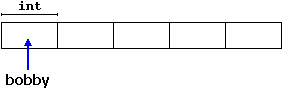# C++ 动态内存

## 操作符new 和new[ ]

pointer = new type

pointer = new type [elements]

int * bobby;
bobby = new int ;int * bobby;
bobby = new int ;
if (bobby == NULL) {
// error assigning memory. Take measures.
};

## 删除操作符delete

delete pointer;

delete [ ] pointer;

// rememb-o-matic
#include ‹iostream.h›
#include ‹stdlib.h›

int main ( ) {
char input ;
int i,n;
long * l;
cout << "How many numbers do you want to type in? ";
cin.getline (input,100); i=atoi (input);
l= new long[i];
if (l == NULL) exit (1);
for (n=0; n<i; n++) {
cout << "Enter number: ";
cin.getline (input,100);
l[n]=atol (input);
}
cout << "You have entered: ";
for (n=0; n<i; n++) {
cout << l[n] << ", ";
delete[] l;
return 0;
}

How many numbers do you want to type in? 5
Enter number : 75
Enter number : 436
Enter number : 1067
Enter number : 8
Enter number : 32
You have entered: 75, 436, 1067, 8, 32,

NULL是C++库中定义的一个常量，专门设计用来指代空指针的。如果这个常量没有被预先定义，你可以自己定以它为0:

#define NULL 0

## 函数malloc

void * malloc (size_t nbytes);

char * ronny;
ronny = (char *) malloc (10);

int * bobby;
bobby = (int *) malloc (5 * sizeof(int));

## 函数calloc

calloc 与malloc 在操作上非常相似，他们主要的区别是在原型上：

void * calloc (size_t nelements, size_t size);

int * bobby;
bobby = (int *) calloc (5, sizeof(int));

malloc 和calloc的另一点不同在于calloc 会将所有的元素初始化为0。

## 函数realloc

void * realloc (void * pointer, size_t size);

## 函数 free

void free (void * pointer);